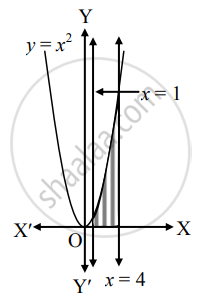# Solve the following : Find the area of the region bounded by y = x2, the X-axis and x = 1, x = 4. - Mathematics and Statistics

Sum

Solve the following :

Find the area of the region bounded by y = x2, the X-axis and x = 1, x = 4.

#### SolutionRequired area = int_1^4y*dx

= int_1^4 x^2*dx

= [x^3/3]_1^4

= (1)/(3)(4^3 - 1^3)

= (1)/(3)(64 - 1)

= (1)/(3)(63)

= 21 sq. units.

Is there an error in this question or solution?
Chapter 7: Applications of Definite Integration - Miscellaneous Exercise 7 [Page 158]

#### APPEARS IN

Balbharati Mathematics and Statistics 1 (Commerce) 12th Standard HSC Maharashtra State Board
Chapter 7 Applications of Definite Integration
Miscellaneous Exercise 7 | Q 4.5 | Page 158
Share